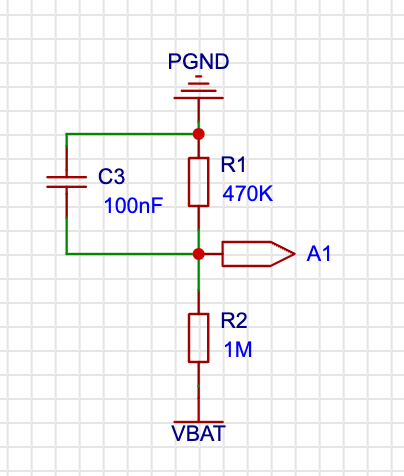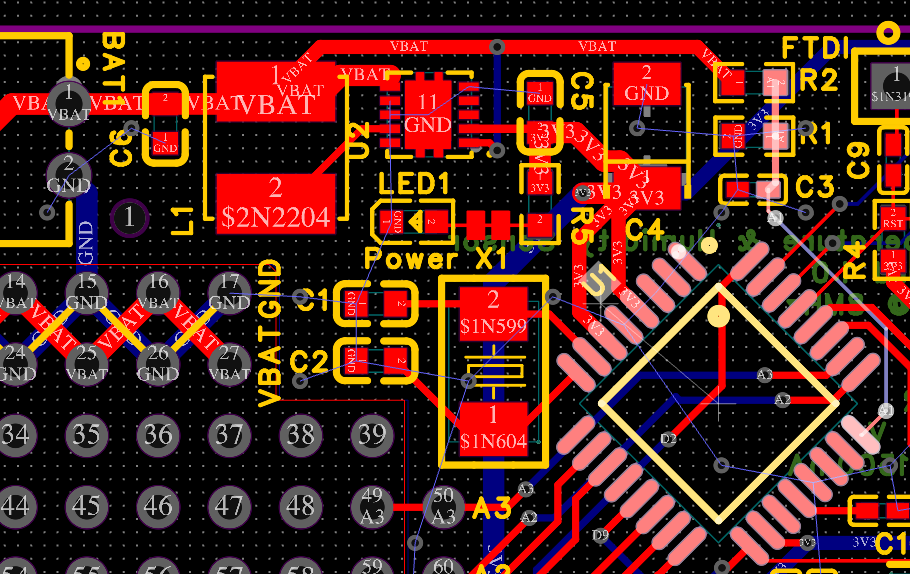# My HW gives me wrong battery voltage

• Hi,
I've built my very first PCB with atmega328p-au. Everything works fine (MCU, sensor, radio) except battery measurement. It always give me 0.4V regardless of what battery is connected (1,5V 1xAA or 3V 2xAA).

``````const float VOLTAGE_DIVIDER_R1 = 1e6;
const float VOLTAGE_DIVIDER_R2 = 470e3;
uint8_t BATTERY_SENSE_PIN = A1;

void setup() {
// use the 1.1 V internal reference
#if defined(__AVR_ATmega2560__)
analogReference(INTERNAL1V1);
#else
analogReference(INTERNAL);
#endif
}
...
// gives me 127

// 3.440426e+00
const float voltageDivider = ((VOLTAGE_DIVIDER_R1+VOLTAGE_DIVIDER_R2)/VOLTAGE_DIVIDER_R2)*1.1;

// 3.363075e-03
const float voltsPerBit = voltageDivider/1023;

// 0.43 V
float batteryV = sensorValue * voltsPerBit; // calculated
``````

R1 = 470K
R2 = 1M
C3 = 100nF
C3 is connected to A1

Any idea what could be wrong?• Your voltage divider math is wrong. It should be of the form

R1/(R1+R2)

However, why even bother with a voltage divider? The analog inputs on the atmega328 can handle the full battery voltage from either of your scenarios.

Although, maybe you have the pin wrong? Or your setting of which board you are using is linking in a different connection? I don't know if the PDIP package would cause it to be a different pin. (I find that hard to believe, but I don't know what else to say.) Cause the reading should obviously change if you're really changing the voltage at the pin that you're reading... Maybe if you can feed direct voltage to the pin temporarily and see if it does anything?

• What @ejiane said. And as a battery saving hint, you even don't need a port pin:

• Set the ADC reference voltage to VCC
• Measure the 1.1V reference
• Calculate VCC

And with MySensors even more easy:

``````hwCPUVoltage()
``````

• @ejlane @eiten problem is that before any calculations `sensorValue` from `analogRead` is 127. So if I use 127 in any formula it's doesn't make and reasonable value.

1000000/(1000000+470000)*127 = 86,3945578231

I took the formula from https://www.mysensors.org/build/battery#external-measurement. R1 and R2 names (not values) are swapped in my schematicBtw, board was meant to be power by 1,2,3 or 4 AA batteries. It's powered by TPS61025DRCR. But I am not sure I did the power management correctly.

• https://github.com/mysensors/MySensors/issues/1489gorbunovav created this issue in mysensors/MySensors

• @igo But in your code you have the wrong calculation. You have (R1+R2)/R2. Whether the names are right or wrong, that calculation is wrong.

But yes, I agree that it is not the root problem, that's why I also talked about trying to find it by checking pin definitions and putting voltage right on the pin.

But what @JeeLet said looks like it's worth checking. Maybe you need to try a loop of a bunch more reads to see if it improves after a bit.

• It's an awfully convoluted way of doing things but suspect your error lies in misunderstanding calculations mixing ints and floats and something screwy with your resistor bridge - It is always worth checking the bridge with a multimeter and raw voltage to verify the expectation.
If you have 3v at the top of your voltage divider, you should be getting 0.9593v applied on the ADC pin which will be read as 892 against the 1.1v internal reference.

The easiest way I found was to define a multiplier needed to derive the raw voltage, in this case (((R1+R2)x1.1)/R2) - For your resistor arrangement the max you can read is 3.4404v before exceeding the 1.1v internal reference.
To reverse the ADC reading of 892 to raw voltage is (892x3.4404)/1023 = 2.9984 v.

Perhaps this is a simpler way to do it

``````float MULTIPLIER= (((1000+470)*1.1)/470);//Resistor bridge values in k and Vref
float batteryV = (sensorValue * MULTIPLIER)/1023.0; //Note the trailing decimal point on the 1023 for calculations involving floats
``````

87

8

7

7

5

1